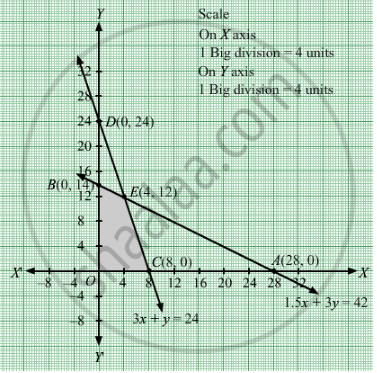# A Factory Makes Tennis Rackets and Cricket Bats. Find the Number of Tennis Rackets and Cricket Bats that the Factory Must Manufacture to Maximum Profit. Make It as an Lpp and Solve It Graphically. - Mathematics

Sum

A factory makes tennis rackets and cricket bats. A tennis racket takes 1.5 hours of machine time and 3 hours of craftman's time in its making while a cricket bat takes 3 hours of machine time and 1 hour of craftman's time. In a day, the factory has the availability of not more than 42 hours of machine time and 24 hours of craftman's time. If the profit on a racket and on a bat is Rs 20 and Rs 10 respectively, find the number of tennis rackets and cricket bats that the factory must manufacture to earn the maximum profit. Make it as an LPP and solve it graphically.

#### Solution

Let x number of tennis rackets and y number of cricket bats were sold.
Number of tennis rackets and cricket balls cannot be negative.
Therefore , $x \geq 0, y \geq 0$

It is given that a tennis racket takes 1.5 hours of machine time and 3 hours of craftman's time in its making while a cricket bat takes 3 hours of machine time and 1 hour of craftman's time.

Also, the factory has the availability of not more than 42 hours of machine time and 24 hours of craftman's time.Therefore,
$1 . 5x + 3y \leq 42$
$3x + y \leq 24$

If the profit on a racket and on a bat is Rs 20 and Rs 10 respectively.Therefore, profit made on tennis rackets and y cricket bats is Rs 20x and Rs 10y respectively.
Total profit = Z = 20x + 10y

The mathematical form of the given LPP is:

Maximize Z = 20x + 10y

Subject to constraints:

$1 . 5x + 3y \leq 42$
$3x + y \leq 24$

$x \geq 0, y \geq 0$

First we will convert inequations into equations as follows:
1.5x + 3y = 42, 3x + y = 24, x = 0 and y = 0

Region represented by 1.5x + 3y  ≤ 42:

The line 1.5x + 3y = 42 meets the coordinate axes at A1(28, 0) and $B_1 \left( 0, 14 \right)$ respectively. By joining these points we obtain the line 1.5x + 3y = 42. Clearly (0,0) satisfies the 1.5x + 3y = 42. So, the region which contains the origin represents the solution set of the inequation 1.5x + 3y  ≤ 42.

Region represented by 3x y ≤ 24:
The line 3x + y = 24 meets the coordinate axes at C1(8, 0) and  $D_1 \left( 0, 24 \right)$ respectively. By joining these points we obtain the line 3x + y = 24. Clearly (0,0) satisfies the inequation 3x y ≤ 24. So,the region which contains the origin represents the solution set of the inequation 3x y ≤ 24.

Region represented by ≥ 0 and y ≥ 0:
Since, every point in the first quadrant satisfies these inequations. So, the first quadrant is the region represented by the inequations x ≥ 0, and y ≥ 0.

The feasible region determined by the system of constraints 1.5x + 3y  ≤ 42, 3x y ≤ 24, x ≥ 0 and y ≥ 0 are as follows.In the above graph, the shaded region is the feasible region.

The corner points are O(0, 0), B1(0, 14), E1(4, 12), and C1(8, 0).

The values of the objective function Z at corner points of the feasible region are given in the following table:

 Corner Points Z = 20x +10y O(0, 0) 0 B1(0, 14) 140 E1(4, 12) 200 ← Maximum C1(8, 0) 160

Clearly, Z is maximum at x = 4 and y = 12 and the maximum value of Z at this point is 200.

Thus, maximum profit is of Rs 200 obtained when 4 tennis rackets and 12 cricket bats were sold.

Concept: Graphical Method of Solving Linear Programming Problems
Is there an error in this question or solution?

#### APPEARS IN

RD Sharma Class 12 Maths
Chapter 30 Linear programming
Exercise 30.4 | Q 40 | Page 55